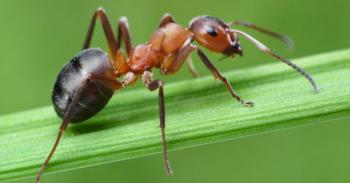Games
Problems
Go Pro!

Twelfth grader Jodi asks, "Hi Professor, if you could help me understand this problem I would highly appreciate it. Problem: An ant starts digging a tunnel. On the 1st day he digs 9m north. The 2nd day he digs 6 m east. The 3rd day he digs 4m south. He continues to dig in this pattern. Question 1: How far will he dig in total? Question 2: How far north from his starting point will he end up?"Hi Jodi, this is definitely a fun problem. I'm going to answer these two questions, and then I'm going to pose two more questions for you to think about.

## Question 1: How far will the ant dig?

Each day the ant digs 2/3 of what he dug the day before. Thus, his total distance is

Eq. 1: D = 9 + 6 + 4 + 8/3 + 16/9 + ...

Now, there are formulas you can use to calculate that sum, and if you're in a Pre-Calculus class (or higher) you probably know those formulas. I'm going to show you how to get that sum without a formula.  I'm going to take the equation and multiply it by 2/3:

Eq. 2: 2/3D = 6 + 4 + 8/3 + 16/9 + 32/27 + ...

Now, if I subtract the second equation from the first one, all but one of the terms on the right hand side of the equation cancel out, and  I get the following:

Eq. 3: D/3 = 9

This leads to D = 27, which is the answer to Question 1. If you want to make sure, pull out a calculator and punch in a bunch of these terms; you'll see that it doesn't take more than 7 or 8 terms for you to start getting close to 27.

## Question 2: How far north from his starting point will he end up?

Before we get started, I'd like to point out that the problem seems just a tiny bit ambiguous. There are a couple ways I could read this:

1. The ant digs North, then East, then South, then repeats this pattern, going North, East, South, North, East, South, etc.
2. The ant digs North, then East, then South, then North, then West (completing the compass pattern) before repeating all four directions.

Strictly speaking, interpretation #1 is the precise reading of the problem, but I have a feeling interpretation #2 was intended, so I'm going to go with that one.

To solve this, we treat a distance south as a negative distance, and we look at the odd numbered terms of the original sequence (because the even numbered terms are east/west motions, and don't affect the north/south distance).

Eq 4: DN = 9 - 4 + 16/9 - 64/81 + ...

This time the common ratio is negative (we know this because the terms are alternating signs). It is -4/9. So you can do the process I did above, multiplying the equation by -4/9 and then subtracting. Or you can use the formula I referred to above, but didn't tell you:

Eq 5: S = a/(1 - r), where a is the first term and r is the ratio.

DN = 9/(1 - (-4/9)) = 9/(13/9) = 81/13

## Question 3: What about the other digging pattern?

We made the assumption that the problem intended for the ant to dig in all four compass directions, but that may not have been the intent. What if the western direction is skipped each time? How does that affect the answer. It definitely makes it more interesting; I think you'll end up needing to treat it as two separate series: DN and DS, and then find the difference.

## Question 4: What's the total displacement?

Displacement is a word we use a lot in Physics. It's different from the concept of distance (which is how far the ant traveled). Displacement is how far (and in what direction) the ant ended up from where he started, and it's a combination of the distance north, and the distance east. To find that answer, you would need to set up a DE equation (distance east), and then do the Pythagorean Theorem on DN and DE to find the hypotenuse, which is the distance from his starting point to his ending point.

Since displacement is a vector (magnitude and direction) you'd also have to do a bit of trig to find the direction in which the ant ended up from his starting point.

Those are problems I'll leave you to contemplate. Thanks for asking!

Professor Puzzler

# Blogs on This SiteReviews and book lists - books we love!The site administrator fields questions from visitors.Like us on Facebook to get updates about new resources